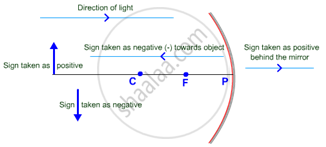# Sign Convention for Reflection by Spherical Mirrors

#### notes

Sign convictions:-

While dealing with the reflection of light by spherical mirrors, we shall follow a set of sign conventions called the New Cartesian Sign Convention. In this convention, the pole (P) of the mirror is taken as the origin. The principal axis of the mirror is taken as the x-axis (X’X) of the coordinate system. The conventions are as follows –

(i) The object is always placed to the left of the mirror. This implies that the light from the object falls on the mirror from the left-hand side.

(ii) All distances parallel to the principal axis are measured from the pole of the mirror.

(iii) All the distances measured to the right of the origin (along + x-axis) are taken as positive while those measured to the left of the origin (along – x-axis) are taken as negative.

(iv) Distances measured perpendicular to and above the principal axis (along + y-axis) are taken as positive.

(v) Distances measured perpendicular to and below the principal axis (along –y-axis) are taken as negative.

Concave mirror:

• Since, object is always placed in front of the mirror hence the sign of object is taken as negative.
• Since, the centre of curvature and focus lie in front of the concave mirror, so signs of radius of curvature and focal length are taken as negative in the case of concave mirror.
• When image is formed in front of the mirror, the distance of image is taken as – (negative) and when image is formed behind the mirror, the distance of image is taken as + (positive).
• Height of image is taken as positive in the case of erect image and taken as negative in the case of inverted image.

Convex mirror:

• Since, object is always placed in front of the mirror hence the sign of object is taken as negative.
• Since, the centre of curvature and focus lies behind the convex mirror, so sign of radius of curvature and focal length are taken as + (positive) in the case of convex mirror.
• In the case of convex mirror, image always formed behind the mirror, thus the distance of image is taken as positive.
• In the case of a convex mirror, always an erect image is formed, thus the height of image is taken as positive.If you would like to contribute notes or other learning material, please submit them using the button below.

### Shaalaa.com

Light Reflection and Refraction part 9 (Sign convention of Sherical Mirror) [00:01:45]
S
0%